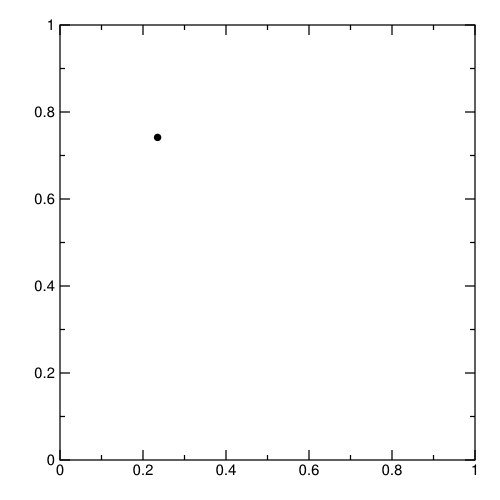Home > Hadrian > Basic clustering models

# Basic clustering models

## Before you begin…

Launch a Python prompt and import the following:

``````Python 2.7.6
>>> import random
>>> import math
>>>
>>> import titus.prettypfa
>>> from titus.genpy import PFAEngine
``````

## The basic form

To use the functions in `model.cluster.*`, declare your clusters as records with a “center” field. (The centers don’t have to be arrays of numbers: they can be arrays of any type `X` as long as you have a metric function that can find distances on `X`.)

``````pfaDocument = titus.prettypfa.jsonNode('''
types:
Cluster = record(Cluster,
center: array(double),
population: int)
input: array(double)
output: Cluster
cells:
clusters(array(Cluster)) = []
action:
model.cluster.closest(input, clusters, metric.simpleEuclidean)
''')
``````

This scoring engine returns the whole cluster object for each match. You could consider adding an “id” (`string`) field and return the id of the closest cluster.

## Producing a model

Since `titus.producer.kmeans` already has an implementation of k-means clustering, let’s build [hierarchical clusters])(https://en.wikipedia.org/wiki/Hierarchical_clustering) (complete linkage).

``````def randomPoint():
return [random.gauss(0, 1), random.gauss(0, 1), random.gauss(0, 1)]

def pointDistance(xpoint, ypoint):
return math.sqrt(sum((xi - yi)**2 for xi, yi in zip(xpoint, ypoint)))

def setDistance(xset, yset):
maxDistance = None
for xpoint in xset:
for ypoint in yset:
d = pointDistance(xpoint, ypoint)
if maxDistance is None or d > maxDistance:
maxDistance = d
return maxDistance

dataset = [randomPoint() for x in xrange(100)]

clusters = [[x] for x in dataset]

DISTANCE_CUTOFF = 3.0
while len(clusters) > 1:
distance, besti, bestj = None, None, None
for i in xrange(len(clusters)):
for j in xrange(i + 1, len(clusters)):
d = setDistance(clusters[i], clusters[j])
if distance is None or d < distance:
distance = d
besti = i
bestj = j
if distance > DISTANCE_CUTOFF:
break
clusters.append(clusters[besti] + clusters[bestj])
del clusters[bestj]
del clusters[besti]
``````

## Insert the model into PFA

The clusters as I’ve defined them aren’t in the right format for my `Cluster` type above, so I’ll have to convert them before inserting them.

``````def makeCluster(list):
center = [0.0] * len(list)
for vector in list:
for i in xrange(len(center)):
center[i] += vector[i] / len(list)
return {"center": center, "population": len(list)}

``````

## Test it!

``````engine, = PFAEngine.fromJson(pfaDocument)

for point in dataset:
print "{0:70s} -> {1}".format(point, engine.action(point))
``````

## Streaming clusters

PFA is ordinarily used for making predictions from a fixed model because it describes a single-pass procedure. (The logic for multiple iterations on a dataset would be contained in the program that runs the PFA, not the PFA itself.) Thus, the usual mode is to perform k-means or hierarchical clustering on a training dataset (which requires iterations) and then apply the resulting model to classify new data, as shown in the sections above.

However, it is also possible to update clusters on the fly on a sliding window. The `model.cluster.*` library contains methods for seeding and updating clusters. The approach is to apply one k-means iteration to a window of data every time the window increments. Most of the data in that window is unchanged from one step to the next, so this procedure is similar to iteration, apart from the old data points that fall off one end of the window and new data points that are added to the other.

The YAML file below describes a PFA engine based on this technique. (The subset of YAML used is equivalent to JSON, but easier to read, and it allows comments.)

``````# Input: get a 2D vector.
input: {type: array, items: double}

# Output: emit the current state of the clusters.
output:
type: array
items:
type: record
name: Cluster
fields:
- {name: center, type: {type: array, items: double}}

# Keep track of the dataset (window of size 100) and the clusters (initially
# empty).
cells:
window:
type: {type: array, items: {type: array, items: double}}
init: []
clusters:
type: {type: array, items: Cluster}
init: []

# For each input vector, apply this action...
method: emit
action:
# First, put the datum into the circular buffer (maintained by the a.cycle
# function).
- cell: window
to:
params: [{x: {type: array, items: {type: array, items: double}}}]
ret: {type: array, items: {type: array, items: double}}
do: {a.cycle: [x, input, 100]}

# Next, update the clusters if the window has more than 100 entries.
- if: {">=": [{a.len: {cell: window}}, 100]}
then:
emit:
cell: clusters
to:
params: [{oldClusters: {type: array, items: Cluster}}]
ret: {type: array, items: Cluster}
do:
if: {"==": [{a.len: oldClusters}, 0]}

# If there are no clusters yet, randomly select three data points
# to use as seeds.
then:
model.cluster.randomSeeds:
- {cell: window}
- 3
- params: [{i: int}, {vec: {type: array, items: double}}]
ret: Cluster
do:
new: {center: vec}
type: Cluster

# If clusters already exist, apply one k-means iteration every time
# the window slides one place. Also, include the old centers in the
# mean with as much weight as the new window (100.0).
else:
model.cluster.kmeansIteration:
- {cell: window}
- oldClusters
- {fcnref: metric.simpleEuclidean}
- params:
- {data: {type: array, items: {type: array, items: double}}}
- {cluster: Cluster}
ret: Cluster
do: {model.cluster.updateMean: [data, cluster, 100.0]}
``````

The plot below shows what this looks like. Black points are data in the windows, which clearly belong to three clusters, and after a burn-in period (100 events), cluster centers (red cross-hairs) are randomly seeded and then updated. The true centers of the clusters are intentionally changed during the run, to show how the scoring engine reacts.